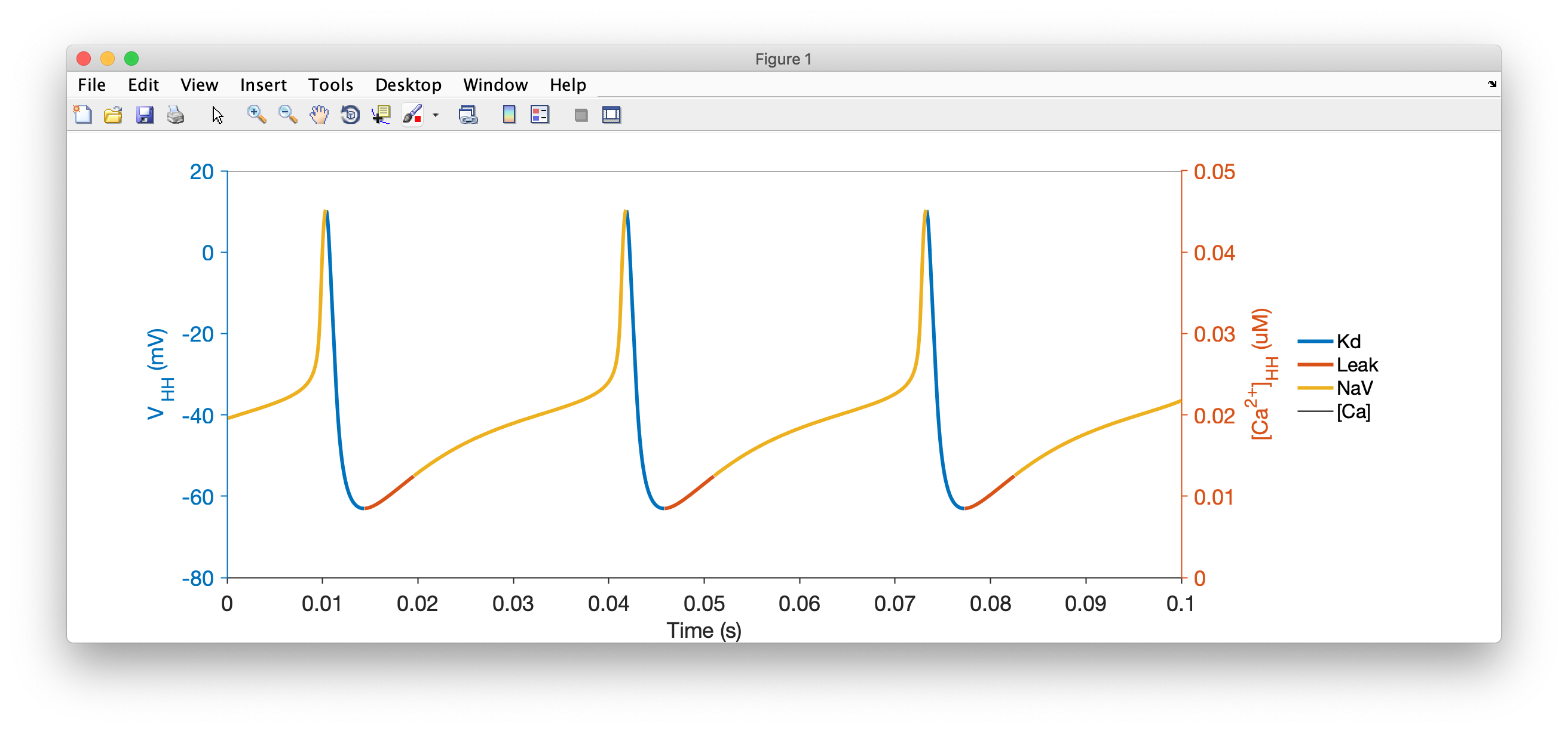In this tutorial, we will walk through the process of creating a model of a single neuron, inserting some conductances in it, and integrating it and viewing its output.

### A high-level view of a neuron¶

A neuron can be approximated by a sphere such that it is well-described by a single membrane voltage, the dynamics of which are governed by populations of ion channels in the cell as illustrated in the schematic below:### Implementing this in xolotl¶

Note that this description of a neuron imposes a natural hierarchy on the things in our model: the soma contains various populations of ion channels. We will create a model in xolotl that looks like this:See that the compartment object contains the three populations of ion channels, just like in our description of the neuron?

To set up this model, the first thing we will do is create a new xolotl object:

x = xolotl;


### Making a compartment¶

By convention, this variable will be called x, though you can call it whatever you want. Now, let's build up out model from the outside in. First, let's make a new compartment and add it:

x.add('compartment','HH','A',.01);


Here, we are creating a new object of type compartment, setting its surface area A to .01 $mm^2$, naming it HH (for Hodgkin-Huxley), and adding it to our xolotl object, all in one line.

If you look at your xolotl object by typing x in the MATLAB prompt, you should see something like this:

 xolotl object with
---------------------
+ HH
---------------------


This tells you that there is only one compartment, and that it's called "HH", and there is nothing in it.

### Adding channels to our compartment¶

A compartment is pretty boring by itself. Let's add some voltage-gated channels to it to give it some excitability (no pun intended).

To do this, we type:

x.HH.add('liu/NaV', 'gbar', 1e3, 'E', 30);


What's going on here? In each line, we are specifying the thing that we want to add to HH, and we are specifying some parameters of the thing we're adding using name value syntax.

Let's break this down by focusing on the first line. We're adding a component that is specified by the string liu/NaV to HH. This is a voltage gated sodium conductance. We're setting its maximal conductance to 1000 uS/mm^2, and its reversal potential to 30mV and adding it to the model. The same logic works for the other two lines.

When we look at our model by typing x, we see:

xolotl object with
---------------------
+ HH
> Kd (g=300, E=-80)
> Leak (g=1, E=-55)
> NaV (g=1000, E=30)
---------------------


Now we see that the compartment HH has three children, representing the three populations of ion channels that we added to it. We can see the maximum conductance (g) and the reversal potentials (E) of each channel type.

That's it! Let's integrate it and see what it does.

### Integrating the model¶

To integrate the model and plot the voltage, we type:

x.t_end = 100; % ms
x.plot;


You should see something like this:Hmm. That's very boring. This neuron isn't doing anything. Let's inject some current into it and see if it does something.

x.I_ext = .2; %nA
x.plotWe see that the neuron now spikes regularly.

What's with the colors?

The voltage trace is coloured by the dominant current at that time. When the voltage is increasing, the color corresponds to the largest inward current. When the voltage is decreasing, the color corresponds to the largest outward current. Here, you can see that the upswing of each action potential is dominated by Sodium currents, while the repolarization is caused by Potassium currents.

### Interacting with the simulation¶

xolotl allows you to manipulate every single parameter in the model and view what effect it has on your model. For example, if we wanted to manipulate the maximal conductances of every channel in this neuron, we can do so using:

x.manipulate('*gbar')


and a window like this should pop up:

Play with the sliders and see how the voltage trace changes.